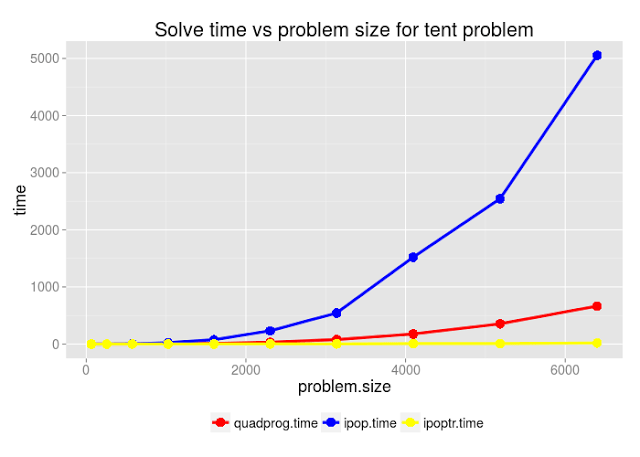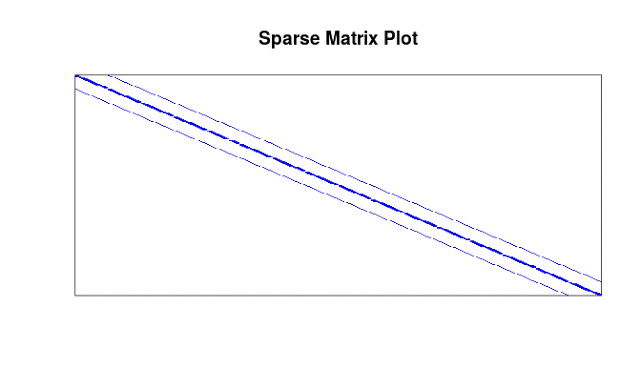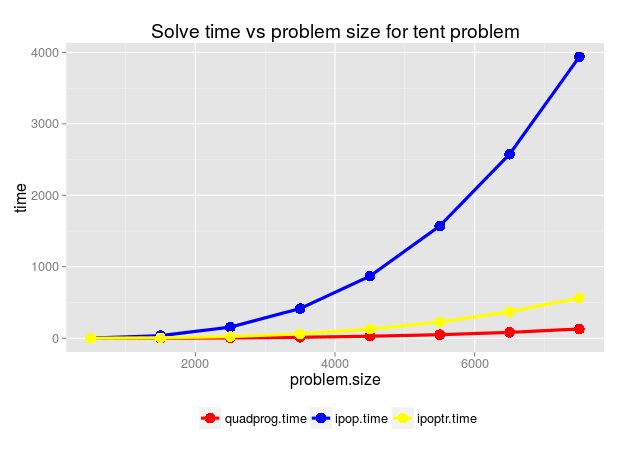# Sparse Quadratic Programming with Ipoptr

This post is a follow up to my last post on quadratic programming facilities in R. A commenter pointed me to the ipoptr project which exposes an R interface to the COIN-OR optimization routine Ipopt. COIN-OR is a suite of optimization utilities implemented in C++ and supported by a back-end of configurable FORTRAN linear system solvers. ipoptr may be a good solution for users wishing to solve sparse nonlinear constrained optimization problems through an R frontend. Some highlights of this solver are:

1. It is a general interior point solver that can handle nonlinear objectives with nonlinear constraints. In particular, no convexity assumptions are required to obtain local solutions.
2. It offers a flexible and lightweight specification of the objective function and a sparse matrix representation for the constraints and other problem data.

In this post, I’ll explain how ipoptr can be applied to solve quadratic programs and I’ll compare the performance of this solver to other quadratic program solvers (quadprog, ipop) available in R. We’ll see that ipoptr is very fast and efficient on large sparse quadratic programs, seemingly an order of magnitude faster than quadprog on the demonstration problem considered in my previous post. Because the Ipopt backend is a bit tricky to install, the last section provides a detailed overview of how I successfully built this solver under Ubuntu Linux.

## The ipoptr interface

Before applying ipoptr to quadratic programming, it may be helpful to present a high-level picture of the ipoptr interface. The following is a summary of the ipoptr interface drawn from the documentation. ipoptr stages and invokes an interior point algorithm from within R to find a local solution of the following constrained optimization problem:
\begin{aligned}\underset{\alpha \in \mathbb{R}^n}{\text{Minimize}}: \qquad & f(x) \\ \text{Subject to:} \qquad & g_L \leq g(x) \leq g_U \\ & x_L \leq x \leq x_U \end{aligned}
where:

• $f:\mathbb{R}^n \to \mathbb{R}$ the objective function is twice continuously differentiable
• $g:\mathbb{R}^n \to \mathbb{R}^m$ defines the constraint set and is twice continuously differentiable
• $x_U$, and $x_L$ are fixed vectors in $\mathbb{R}^n$
• $g_U$, and $g_L$ are fixed vectors in $\mathbb{R}^m$

Note that the solver does not need to make any assumptions about the convexity of either $f$ or $g$. The solver makes use of the following ingredients to find a solution:

1. An initial guess $x_0$ for the solution
2. The objective function gradient $\nabla f$
3. the Jacobian of the constraint map $J_g$
4. Optionally, the Hessian of the problem Lagrangian in the form: $$H(x, \sigma_f, \vec{\lambda}) = \sigma_f \nabla^2 f(x) + \sum_{i=1}^m \lambda_i \nabla^2 g_i(x).$$

When $n$ is large, the dense matrix representations of $g$, $\nabla f$, and $J_g$ will have a substantial footprint. In application problems, however, the Jacobian and/or the Hessian object will often be sparse.  For efficiency, ipoptr utilizes a sparse matrix representation for $H$ and $J_g$.  This format is defined as follows. Let $A_{\mathrm{values}}$ be the list of all non-zero values in $A$ (read into the list from left to right along the rows of $A$).  Then let $A_{\mathrm{mask}}$ be a list of lists, where the list at position $i$ in $A_{\mathrm{mask}}$ provides the column indexes of the nonzero elements in the $i$-th row of $A$. Then $(A_{\mathrm{values}}$, $A_{\mathrm{mask}})$ is a sparse representation of $A$.

Generally, it could be quite difficult to come up with the explicit representations of the Jacobian and Hessian that ipoptr consumes. Although the Hessian is an optional argument, including it can significantly improve convergence behaviour (try taking the Hessian arguments out in the examples below). For a quadratic program, however, it is simple to compute the Jacobian and Hessian directly. We’ll do this in the next section and then provide an R wrapper function that can transform any symmetric positive definite quadratic program in standard matrix form into a quadratic program that can be solved by ipoptr.

## Solving Quadratic Programs with ipoptr

\begin{aligned} \underset{\alpha \in \mathbb{R}^n}{\text{Minimize}}: \qquad & f(x) = -d^Tx + \frac{1}{2}x^T D x \\ \text{Subject to:} \qquad & g(x) = Ax \leq b \end{aligned}
where

• $D$ is an $n \times n$ matrix (which we’ll assume is symmetric)
• $A$ is an $m \times n$ matrix.
• $d$ is a fixed vector in $\mathbb{R}^n$ and $b$ is a fixed vector in $\mathbb{R}^m$.

Then using some basic identities of vector calculus and the symmetry of $D$:
\begin{aligned} \nabla f(x) &= -d + \frac{1}{2}(D^T + D) x = -d + Dx \\ \nabla^2 f(x) &= D \\ J_g(x) &= A^T \\ \nabla^2 g_i(x) &= 0 \mbox{ for all } i \\ H(x, \sigma_f, \vec{\lambda}) &= \sigma_f D. \end{aligned}
Because $D$ is positive definite, the global minimizer $\tilde{x}$ of $f$ can be computed as:
$$\nabla f(\tilde{x}) = 0 \rightarrow \tilde{x} = D^{-1} d.$$
Goldfarb and Idnani, whose QP algorithm is implemented in the quadprog package, use the global minimizer $\tilde{x}$ as the initial point for their interior point primal/dual method. This choice is based on empirical evidence cited by the authors that this selection can substantially reduce the number of iterations required to find a solution. We’ll follow suit with ipoptr by setting $x_0 =\tilde{x}$ for our initial guess.

The following R function uses these facts to solve a quadratic program in standard form with ipoptr:

## Performance Comparison

In my previous post, I compared the performance of quadprog and kernlab’s ipop solvers on the circus tent demonstration problem. For this post, I repeated the same experiment with the ipoptr solver and the results were very impressive. Ipoptr was substantially faster at solving this sparse demonstration problem than quadprog. Here are the solve times I obtained:problem.size quadprog.time ipop.time ipoptr.time
1            64         0.002     0.024       0.010
2           256         0.048     0.710       0.034
3           576         0.518     4.759       0.126
4          1024         2.903    23.430       0.414
5          1600        10.815    77.708       0.855
6          2304        31.899   232.355       1.819
7          3136        79.749   544.193       3.318
8          4096       176.743  1521.225       8.297
9          5184       354.839  2543.754       9.039
10         6400       664.804  5053.922      19.551

Here is the experiment code:

It’s not so surprising that the ipoptr package is substantially faster in this example because the matrix Dmat that defines the objective function for the tent problem is very sparse. Quadprog requires dense matrix representations to describe the QP that we wish to solve, while in ipoptr we need only specify the functional form the quadratic objective function. Here is the plot of Dmat’s sparsity structure:To investigate the performance of ipoptr on dense QPs, I generated some random positive definite QPs of differing sizes and compared solve times against the solver from quadprog and the ipop solver from the kernlab package. In this dense problem case, quadprog appears to have a significant advantage over ipoptr. Still, ipoptr shows solid performance and is considerably faster than the pure R implementation of the interior point solver found in kernlab’s ipop.problem.size quadprog.time ipop.time ipoptr.time
1          500         0.068     1.942       0.294
2         1500         0.909    35.352       5.374
3         2500         4.598   155.138      23.160
4         3500        13.186   414.762      60.888
5         4500        28.419   868.141     126.278
6         5500        49.608  1570.674     226.914
7         6500        81.756  2574.962     369.469
8         7500       128.451  3937.629     563.236

Here is the code for the randomly generated QP experiment:

In this post, we looked at how the ipoptr interface can be used to solve quadratic programming problems and compared the Ipopt solver to other quadratic program solvers available in R. We found:

• ipoptr is very efficient and well-suited for solving large, sparse quadratic programs, even outperforming the quadprog package solver in this case.
• However, for dense symmetric positive definite QPs, quadprog is much faster than ipoptr.
• Both solvers are substantially faster than the pure R interior point solver ipop from the kernlab package.

• For the experiments in this post, Ipopt was configured to use the MUMPS linear solver. It is possible to configure Ipopt to use other linear solvers and this could have an impact on performance.
• Initializing Ipopt with $x_0$ as the global minimum of the objective function (as done internally in the quadprog package) seems to be somewhat beneficial for reducing runtimes. This effect should be investigated more carefully, however.
• The timings presented above do not include the time required to translate from a QP specified in matrix format (e.g. Dmat, Amat, etc.) to the input format required by ipoptr. In practice, however, this step is rather expensive and users will probably want to generate the ipoptr input data directly instead of forming dense matrix inputs and then converting to the ipoptr format. The specifics of how this should be done will depend on the particular problem which the user is trying to solve.

## Installing ipoptr for Ubuntu Linux

Because of complex licensing issues in COIN-OR suite and its many dependencies, it’s not possible to bundle the backend ipopt solver and its R interface into a simple package installable directly from R. Thus, installing ipoptr won’t be as easy as installing most other R pacakges. Fortunately, the CoinOpt documentation and the R interface documentation are fairly complete.

After a bit of work, I was able to install everything on a Ubuntu-Linux box using the following steps.

#  Change into directory where you want to put the ipoptr source and build path
cd ~/projects/

# install dependencies, including latest blas and lapack.
sudo apt-get update
sudo apt-get install gcc g++ gfortran subversion patch wget
sudo apt-get install libblas3 liblapack3

# get the project -- note, will need to accept SSL cert
svn co https://projects.coin-or.org/svn/Ipopt/stable/3.12 CoinIpopt

# get and build Mumps linear solver
# more than once, the ./get.mumps script has failed for me with a server side network error.
# if that happens, wait a bit and run again.
cd CoinIpopt/ThirdParty/Mumps
./get.Mumps
cd ../..

# build and install Ipopt; it's a good idea to inspect the output of make test
# With this setup, AMPL test will fail (ok) but all the other tests should pass.
mkdir build
cd build
../configure
make
make test
make install

# Move RInterface Makevars from build to src directory
cp Ipopt/contrib/RInterface/src/Makevars ../Ipopt/contrib/RInterface/src

Now, we can fire up R and install the package from source using:

install.packages("~/projects/CoinIpopt/Ipopt/contrib/RInterface",
repos=NULL, type="source")